SSC CGL Previous Year Questions: Trigonometry - 3

# SSC CGL Previous Year Questions: Trigonometry - 3 - SSC CGL

Test Description

## 40 Questions MCQ Test SSC CGL (Tier - 1) - Previous Year Papers (Topic Wise) - SSC CGL Previous Year Questions: Trigonometry - 3

SSC CGL Previous Year Questions: Trigonometry - 3 for SSC CGL 2023 is part of SSC CGL (Tier - 1) - Previous Year Papers (Topic Wise) preparation. The SSC CGL Previous Year Questions: Trigonometry - 3 questions and answers have been prepared according to the SSC CGL exam syllabus.The SSC CGL Previous Year Questions: Trigonometry - 3 MCQs are made for SSC CGL 2023 Exam. Find important definitions, questions, notes, meanings, examples, exercises, MCQs and online tests for SSC CGL Previous Year Questions: Trigonometry - 3 below.
Solutions of SSC CGL Previous Year Questions: Trigonometry - 3 questions in English are available as part of our SSC CGL (Tier - 1) - Previous Year Papers (Topic Wise) for SSC CGL & SSC CGL Previous Year Questions: Trigonometry - 3 solutions in Hindi for SSC CGL (Tier - 1) - Previous Year Papers (Topic Wise) course. Download more important topics, notes, lectures and mock test series for SSC CGL Exam by signing up for free. Attempt SSC CGL Previous Year Questions: Trigonometry - 3 | 40 questions in 40 minutes | Mock test for SSC CGL preparation | Free important questions MCQ to study SSC CGL (Tier - 1) - Previous Year Papers (Topic Wise) for SSC CGL Exam | Download free PDF with solutions
 1 Crore+ students have signed up on EduRev. Have you?
SSC CGL Previous Year Questions: Trigonometry - 3 - Question 1

### A kite is flying at the height of 75 m from the ground. The string makes an angle q (where cot θ = 8/15) with the level ground. Assuming that there is no slack in the string, the length of the string is equal to:    (SSC CGL 1st Sit. 2015)

Detailed Solution for SSC CGL Previous Year Questions: Trigonometry - 3 - Question 1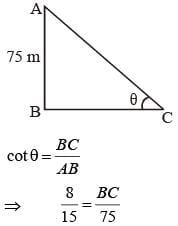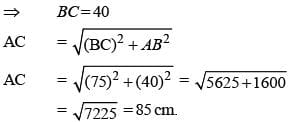SSC CGL Previous Year Questions: Trigonometry - 3 - Question 2

### If 5 sin θ = 3, the numerical value of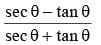(SSC CGL 1st Sit. 2015)

Detailed Solution for SSC CGL Previous Year Questions: Trigonometry - 3 - Question 2

5 sin θ = 3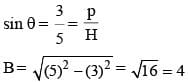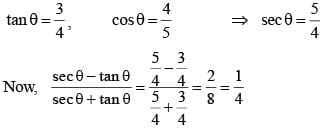SSC CGL Previous Year Questions: Trigonometry - 3 - Question 3

### If q be acute angle and tan (4θ – 50°) = cot (50° – θ), then the value of θ in degrees is:    (SSC CGL 1st Sit. 2015)

Detailed Solution for SSC CGL Previous Year Questions: Trigonometry - 3 - Question 3

tan(4θ - 50 ° ) = cot(50 °- θ)
⇒ tan (4θ – 50°) = tan (90 – (50° – θ))
⇒ 4θ – 50° = 90 – 50° + θ
⇒ 3θ = 90° ; θ = 30°.

SSC CGL Previous Year Questions: Trigonometry - 3 - Question 4

The value of sin222° + sin268° + cot230° is    (SSC CGL 1st Sit. 2015)

Detailed Solution for SSC CGL Previous Year Questions: Trigonometry - 3 - Question 4

sin2 22°+ sin2 68°+ cot2 ​​​​​​​30°
= sin2 ​​​​​​​ (90°- 68)°+ sin2 ​​​​​​​ 68°+ cot2 ​​​​​​​ 30°
= cos2 ​​​​​​​ 68°+ sin2 68°+ cot30°
= 1 + (√3)2 = 1 + 3 = 4

SSC CGL Previous Year Questions: Trigonometry - 3 - Question 5

If the sum and difference of two angles are 22/9 radian and 36° respectively, then the value of smaller angle in degree taking the value of π as 22/ 7 is:   (SSC CGL 1st Sit. 2015)

Detailed Solution for SSC CGL Previous Year Questions: Trigonometry - 3 - Question 5

Let angles are x and y rad.
x – y = 36° ... (i)
x + y = 22/9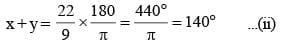Now, on solving each (i) and (ii), we get
x = 88°, and y = 52°
So, smaller angle = 52°

SSC CGL Previous Year Questions: Trigonometry - 3 - Question 6

The minimum  value of 2 sin2 θ + 3 cos2 θ is    (SSC CGL 1st Sit. 2015)

Detailed Solution for SSC CGL Previous Year Questions: Trigonometry - 3 - Question 6

Given that
2sin2θ + 3cos2θ
= 2(1 – cos2θ) + 3cos2θ = 2 – 2cos2θ + 3 cos2θ
= 2 + cos2θ
For minimum value cosθ = 0
Minimum value of 2sin2θ + 3 cos2θ = 2

SSC CGL Previous Year Questions: Trigonometry - 3 - Question 7

A 10 m long ladder is placed against a wall. It is inclined at an angle of 30° to the ground.  The distance (in m) of the foot of the ladder from the wall is (Given √3 = 1.732)    (SSC CGL 1st Sit. 2015)

Detailed Solution for SSC CGL Previous Year Questions: Trigonometry - 3 - Question 7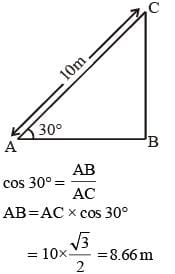SSC CGL Previous Year Questions: Trigonometry - 3 - Question 8

If x cos θ – sin θ = 1, then x2 – (1 + x2) sin θ equals    (SSC CGL 1st Sit. 2015)

Detailed Solution for SSC CGL Previous Year Questions: Trigonometry - 3 - Question 8

x cos θ – sin θ = 1
x cos θ = 1 + sin θ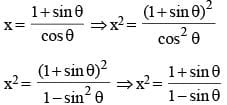⇒ x2 (1 – sin θ) = (1 + sin θ)
x2 – x2  sin θ = 1 + sin θ
x2 – x2 sin θ –1 – sin θ = 0
x2 – (x2 + 1) sin θ = 1

SSC CGL Previous Year Questions: Trigonometry - 3 - Question 9

The numerical value of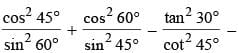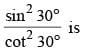(SSC CGL 1st Sit. 2015)

Detailed Solution for SSC CGL Previous Year Questions: Trigonometry - 3 - Question 9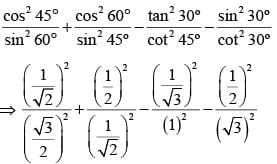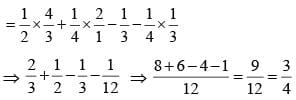SSC CGL Previous Year Questions: Trigonometry - 3 - Question 10

Find the value of tan 4° tan 43° tan 47° tan 86°    (SSC CGL 1st Sit. 2015)

Detailed Solution for SSC CGL Previous Year Questions: Trigonometry - 3 - Question 10

Tan 4° tan 43° tan47° tan 86°
Tan (90° – 86°) × tan (90° – 47°) × tan 47° × tan 86°
= cot 86° × cot 47° × tan 47° × tan 86°
= 1

SSC CGL Previous Year Questions: Trigonometry - 3 - Question 11

The maximum value of sin4θ + cos4θ is    (SSC CGL 1st Sit. 2015)

Detailed Solution for SSC CGL Previous Year Questions: Trigonometry - 3 - Question 11

The maximum value of sin4θ + cos4θ is 1.

SSC CGL Previous Year Questions: Trigonometry - 3 - Question 12

If α + β  = 90° then the expression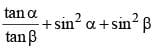is equal to:    (SSC CHSL 2015)

Detailed Solution for SSC CGL Previous Year Questions: Trigonometry - 3 - Question 12

α + β  = 90° ⇒ β = 90° – α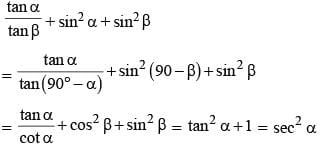SSC CGL Previous Year Questions: Trigonometry - 3 - Question 13

If sin 2θ = √3/2 then the value of sin 3θ is equal to: (take (0° ≤ θ ≤ 90°)    (SSC CHSL 2015)

Detailed Solution for SSC CGL Previous Year Questions: Trigonometry - 3 - Question 13

sin 2θ = √3/2 = sin 60°
⇒ 2θ = 60°,
θ = 30°
sin 3θ = sin 3 (30°) = 90° = sin 90° = 1

SSC CGL Previous Year Questions: Trigonometry - 3 - Question 14

If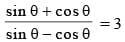then the value of sin2θ is:   (SSC CHSL 2015)

Detailed Solution for SSC CGL Previous Year Questions: Trigonometry - 3 - Question 14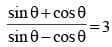By componendo and dividendo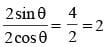So, tan θ = 2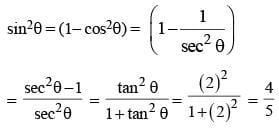SSC CGL Previous Year Questions: Trigonometry - 3 - Question 15

Value of the expression: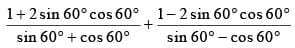(SSC CHSL 2015)

Detailed Solution for SSC CGL Previous Year Questions: Trigonometry - 3 - Question 15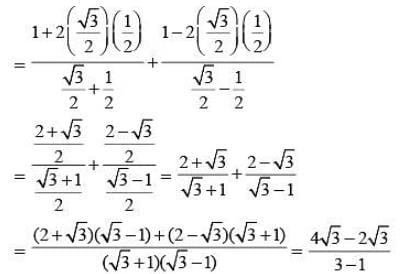= √3/2

SSC CGL Previous Year Questions: Trigonometry - 3 - Question 16

The value of x in the equation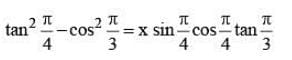(SSC CHSL 2015)

Detailed Solution for SSC CGL Previous Year Questions: Trigonometry - 3 - Question 16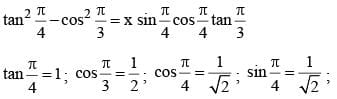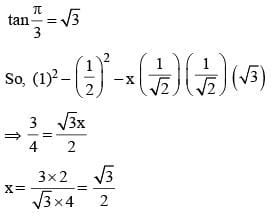SSC CGL Previous Year Questions: Trigonometry - 3 - Question 17

From two points on the ground and lying on a straight line through the foot of a pillar, the two angles of elevation of the top of the pillar are complementary to each other. If the distances of the two points from the foot of the pillar are 12 metres and 27 metres and the two points lie on the same side of the pillar, then the height (in metres) of the pillar is:    (SSC Sub. Ins. 2015)

Detailed Solution for SSC CGL Previous Year Questions: Trigonometry - 3 - Question 17

Let height of the pillar is h m
In ΔACD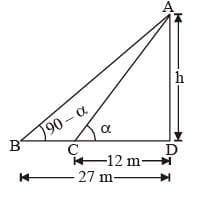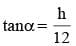In ΔABD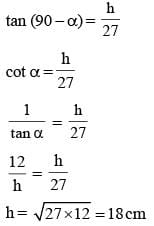SSC CGL Previous Year Questions: Trigonometry - 3 - Question 18

If sin θ + sin2 θ =1, then the value of cos2 θ + cos4 θ is:    (SSC Sub. Ins. 2015)

Detailed Solution for SSC CGL Previous Year Questions: Trigonometry - 3 - Question 18

sinθ + sin2θ = 1
⇒ sinθ = 1 – sin2θ
⇒ sinθ = cos2θ
Squaring both sides
⇒ sin2θ = cos4θ
⇒ 1 – cos2θ = cos4θ
⇒ cos2θ + cos4θ = 1

SSC CGL Previous Year Questions: Trigonometry - 3 - Question 19

If α is an acute angle and 2sin α + 15cos2 α = 7, then the value of cot α is:   (SSC Sub. Ins. 2015)

Detailed Solution for SSC CGL Previous Year Questions: Trigonometry - 3 - Question 19

2sin α + 15cos2 α = 7 where a is acute angle
⇒ 2 sinα + 15 (1 – sin2α) = 7
⇒ 2 sinα + 15 – 15 sin2α – 7 = 0
⇒ – 15 sin2α + 2 sinα + 8 = 0
⇒ 15 sin2α – 2 sinα – 8 = 0
Let sinα = t
So 15t2 – 2t – 8 = 0
⇒ 15t2 – 12t + 10t – 8 = 0
⇒ 3t (5t – 4) + 2 (5t – 4) = 0
⇒ 5t – 4 = 0; 3t + 2 = 0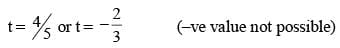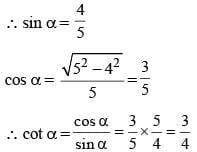SSC CGL Previous Year Questions: Trigonometry - 3 - Question 20

The shadow of a tower standing on a level plane is found to be 40m longer when the sun’s altitude is 45° than when it is 60°. The height of the tower is:    (SSC Sub. Ins. 2015)

Detailed Solution for SSC CGL Previous Year Questions: Trigonometry - 3 - Question 20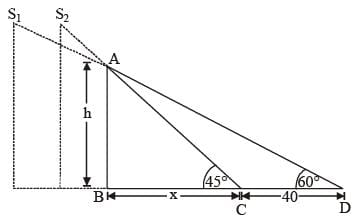Let S1 and S2 be the two different positions of sun and AB is the tower
In ΔABC
tan 45° = h/x  (∵ tan 45° = 1)
h = x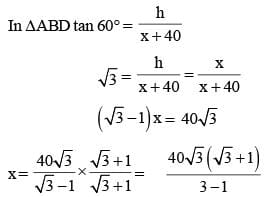= 20√3 (√3 + 1) = 20(3 + √3)m

SSC CGL Previous Year Questions: Trigonometry - 3 - Question 21

The length of the shadow of a vertical tower on level ground increases by 10 metres when the altitude of the sun changes from 45° to 30°. Then the height of the tower is    (SSC CHSL 2014)

Detailed Solution for SSC CGL Previous Year Questions: Trigonometry - 3 - Question 21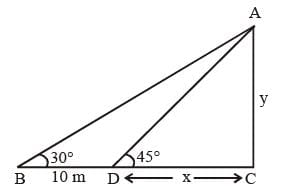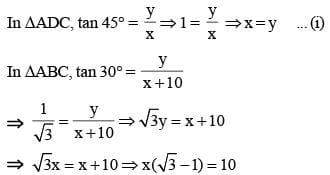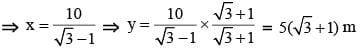SSC CGL Previous Year Questions: Trigonometry - 3 - Question 22

If sin θ + cos θ = √2 cos θ, then the value of cot θ is    (SSC CGL 2014)

Detailed Solution for SSC CGL Previous Year Questions: Trigonometry - 3 - Question 22

sin θ + cos θ = √2 cos θ
sinθ = (√2 - 1) cos θ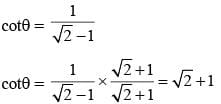SSC CGL Previous Year Questions: Trigonometry - 3 - Question 23

If cos α + sec α = √3, then the value of cos3 α + sec3 α is    (SSC CGL 2014)

Detailed Solution for SSC CGL Previous Year Questions: Trigonometry - 3 - Question 23

cos α + sec α = √3
taking cube both sides
cos3α + sec3 α + 3 cosα secα (cosα + secα)
= 3√3
cos3α + sec3α + 3√3 = 3√3
cos3α + sec3α = 0

SSC CGL Previous Year Questions: Trigonometry - 3 - Question 24

If θ is a positive acute angle and cosec θ + cot θ = √3, then the value of cosec θ is   (SSC CGL 2014)

Detailed Solution for SSC CGL Previous Year Questions: Trigonometry - 3 - Question 24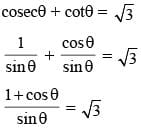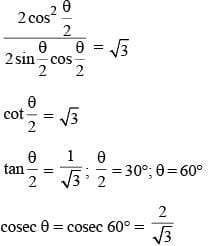SSC CGL Previous Year Questions: Trigonometry - 3 - Question 25

If sin 17° = x/y then sec 17° – sin 73° is equal to    (SSC CGL 2014)

Detailed Solution for SSC CGL Previous Year Questions: Trigonometry - 3 - Question 25

sin17° = x/y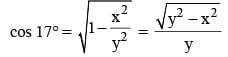sec 17° – sin 73° = sec 17° – cos 17°

SSC CGL Previous Year Questions: Trigonometry - 3 - Question 26

The shadow of a tower standing on a level plane is found to be 30 m longer when the Sun's altitude changes from 60° to 45°. The height of the tower is    (SSC CGL 2014)

Detailed Solution for SSC CGL Previous Year Questions: Trigonometry - 3 - Question 26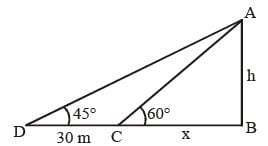In ΔABC, tan60° = h/x
x = h/√3...(1)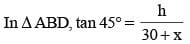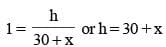Putting value of x from (1)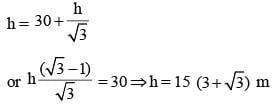SSC CGL Previous Year Questions: Trigonometry - 3 - Question 27

The value of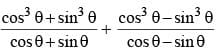is equal to    (SSC CGL 2014)

Detailed Solution for SSC CGL Previous Year Questions: Trigonometry - 3 - Question 27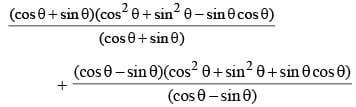= 2 cos2 θ + 2sin2 θ – sin θ cos θ + sinθ cosθ = 2

SSC CGL Previous Year Questions: Trigonometry - 3 - Question 28

The value of sin21° + sin22° + sin23° + .... + sin289° is    (SSC CGL 2014)

Detailed Solution for SSC CGL Previous Year Questions: Trigonometry - 3 - Question 28

(sin2 1° + sin2 89°) + (sin2 2° + sin2 88°) + ... + (sin2 44° + sin2 48°) + sin2 45°
= (sin2 1° + cos2 1°) + (sin2 2° + cos2 2°) + ... + (sin2 44° + cos2 44°) + sin2 45°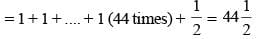SSC CGL Previous Year Questions: Trigonometry - 3 - Question 29

If (r cosθ - √3)2 + (r sinθ – 1)2 = 0, then the value of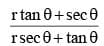is equal to   (SSC CHSL 2014)

Detailed Solution for SSC CGL Previous Year Questions: Trigonometry - 3 - Question 29

(r cosθ - √3)2 + (r sinθ – 1)2 = 0
On comparing, we get
(r cos θ – 3 )2 = 0 ⇒ r cos θ = 3 ... (i)
and (r sin θ – 1)2 = 1 ⇒ r sin θ = 1 ... (ii)
On dividing (ii) from (i), we get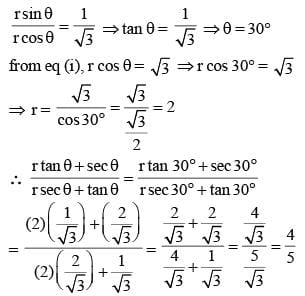SSC CGL Previous Year Questions: Trigonometry - 3 - Question 30

If θ is a positive acute angle and 4 cos2 θ – 4 cos θ + 1 = 0, then the value of tan (θ – 15°) is equal to    (SSC CHSL 2014)

Detailed Solution for SSC CGL Previous Year Questions: Trigonometry - 3 - Question 30

4 cos2θ – 4 cosθ + 1 = 0
(2 cosθ – 1)2 = 0
or, 2 cosθ = 1
⇒ cosθ = 1/2  ; θ = 60°
Hence, the value of tan (θ – 15°) = tan (60° – 15°)
= tan 45° = 1

SSC CGL Previous Year Questions: Trigonometry - 3 - Question 31

The value of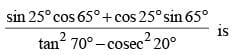(SSC CHSL 2014)

Detailed Solution for SSC CGL Previous Year Questions: Trigonometry - 3 - Question 31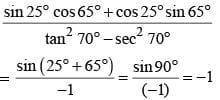SSC CGL Previous Year Questions: Trigonometry - 3 - Question 32

If x = a sin θ and y = b tan θ then prove that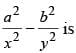(SSC Sub. Ins. 2014)

Detailed Solution for SSC CGL Previous Year Questions: Trigonometry - 3 - Question 32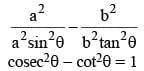SSC CGL Previous Year Questions: Trigonometry - 3 - Question 33

From the top of a light-house at a height 20 metres above sea-level, the angle of depression of a ship is 30°. The distance of the ship from the foot of the light-house is    (SSC Sub. Ins. 2014)

Detailed Solution for SSC CGL Previous Year Questions: Trigonometry - 3 - Question 33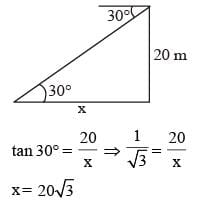SSC CGL Previous Year Questions: Trigonometry - 3 - Question 34

A kite is flying at a height of 50 metre. If the length of string is 100 metre then the inclination of string to the horizontal ground in degree measure is    (SSC Sub. Ins. 2014)

Detailed Solution for SSC CGL Previous Year Questions: Trigonometry - 3 - Question 34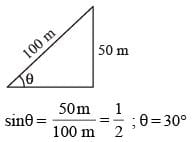SSC CGL Previous Year Questions: Trigonometry - 3 - Question 35

The simplest value of sin2x + 2 tan2x – 2 sec2 x + cos2 x is   (SSC Sub. Ins. 2014)

Detailed Solution for SSC CGL Previous Year Questions: Trigonometry - 3 - Question 35

sin2x + cos2x – 2(sec2x –tan2x)
1 – 2(1) = –1

SSC CGL Previous Year Questions: Trigonometry - 3 - Question 36

If 0 ≤ θ ≤ π/2 and sec2 θ + tan2 θ = 7, then θ is            (SSC Sub. Ins. 2014)

Detailed Solution for SSC CGL Previous Year Questions: Trigonometry - 3 - Question 36

sec2θ + tan2θ = 7
1 + tan2θ + tan2θ = 7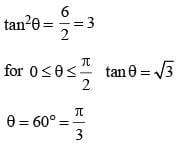SSC CGL Previous Year Questions: Trigonometry - 3 - Question 37

The value of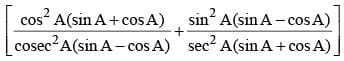(sec2 A – cosec2 A)    (SSC CGL 1st Sit. 2013)

Detailed Solution for SSC CGL Previous Year Questions: Trigonometry - 3 - Question 37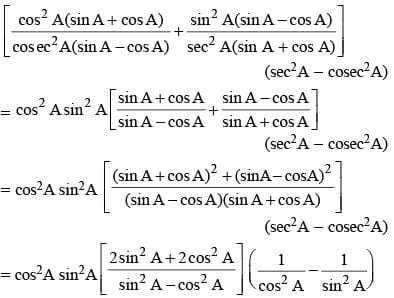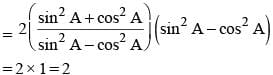SSC CGL Previous Year Questions: Trigonometry - 3 - Question 38

sin2θ – 3 sinθ + 2 = 0 will be true if    (SSC CGL 1st Sit. 2013)

Detailed Solution for SSC CGL Previous Year Questions: Trigonometry - 3 - Question 38

sin2 θ – 3 sin θ + 2 = 0
⇒ sin2 θ – 2 sin θ – sin θ + 2 = 0
⇒ sin θ (sinθ – 2) – 1 (sin θ – 2) = 0
⇒ (sin θ – 1) (sin θ – 2) = 0
⇒ sin θ = 1 = sin 90°
⇒ θ = 90° and sin θ ≠ 2

SSC CGL Previous Year Questions: Trigonometry - 3 - Question 39

The eliminant of θ from x cos θ – y sin θ = 2 and x sin θ + y cos θ = 4 will give    (SSC CGL 1st Sit. 2013)

Detailed Solution for SSC CGL Previous Year Questions: Trigonometry - 3 - Question 39

x cos θ – y sin θ = 2
x sin θ + y cos θ = 4
On squaring both the equations and adding
x2cos2 θ + y2 sin2 θ – 2xy sin θ. cos θ + x2sin2 θ + y2 cos2θ + 2xy sinθ . cosθ
= 4 + 16
⇒ x2 (cos2 θ + sin2 θ) + y2 (sin2 θ + cos2 θ) = 20
⇒ x2 + y2 = 20

SSC CGL Previous Year Questions: Trigonometry - 3 - Question 40

If tan θ + cot θ = 2, then the value of tan2θ + cot2θ is    (SSC CGL 1st Sit. 2013)

Detailed Solution for SSC CGL Previous Year Questions: Trigonometry - 3 - Question 40

tan θ + cot θ = 2
On squaring both sides,
(tan θ + cot θ)2 = 4
⇒ tan2θ + cot2θ + 2tanθ cotθ = 4
⇒ tan2θ + cot2θ = 4 – 2 = 2     [tan θ. cot θ = 1]

## SSC CGL (Tier - 1) - Previous Year Papers (Topic Wise)

250 tests
Information about SSC CGL Previous Year Questions: Trigonometry - 3 Page
In this test you can find the Exam questions for SSC CGL Previous Year Questions: Trigonometry - 3 solved & explained in the simplest way possible. Besides giving Questions and answers for SSC CGL Previous Year Questions: Trigonometry - 3, EduRev gives you an ample number of Online tests for practice

250 tests